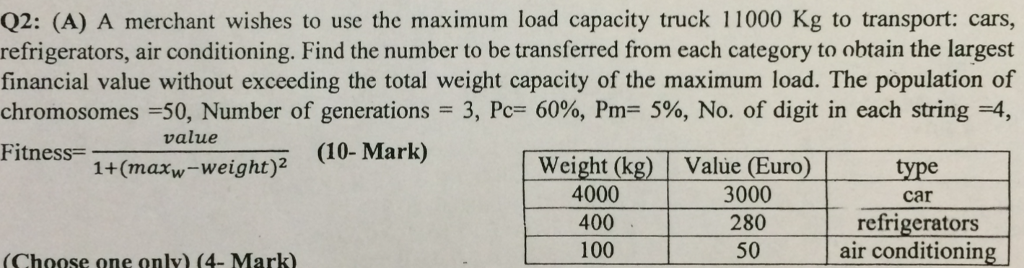# Question & Answer: A merchant wishes to use the maximum load capacity truck 11000 Kg to transport: cars, refrigerators, air conditioning. Find the n…..

Genetic AlgorithmA merchant wishes to use the maximum load capacity truck 11000 Kg to transport: cars, refrigerators, air conditioning. Find the number to be transferred from each category to obtain the largest financial value without exceeding the total weight capacity of the maximum load. The population of chromosomes = 50, Number of generations = 3, Pc = 60%, Pm = 5%, No. of digit in each string = 4, Fitness = value/1 + (max_w – weight)^2 (Choose one only)

Don't use plagiarized sources. Get Your Custom Essay on
Question & Answer: A merchant wishes to use the maximum load capacity truck 11000 Kg to transport: cars, refrigerators, air conditioning. Find the n…..
GET AN ESSAY WRITTEN FOR YOU FROM AS LOW AS \$13/PAGE

Maximum Load Capacity : 11000 kg

Weight(kg) Value(Euro) Type

4000 3000 Car

400 280 Refridgerators

100 50 Air Conditioners

First we will divide the maximum load with weight of that appliance which has maximum value.

-> 11000/4000 = 2 and 3000(remainder)

Value : 2*3000 = 6000

Next we will divide the remainder with the weight of appliance having next maximum value.

-> 3000/400 = 7 and 200(remainder)

Value = 6000 + 7*280 = 6000 + 1960 = 7960

Finally we will divide the remainder with the weight of appliance having least value.

-> 200/100 = 2

Value = 7960 + 2*50 = 8060

so the number of appliances will be 2 Car’s, 7 Refridgerators and 2 Air Conditioners having total weight of 11000 kg and value of 8060.

Generic Algorithm :

MaxWeight

initialize max with maximum weight

initialize weight array with all the weights

initialize value array with corresponding values

sort the value array in descending order if not sorted along with corresponding weight array

initialize curr_wt to 0

initialize i to 0

initialize max_value to 0

initialize no_appliance to 0

while max – curr_wt > 0 loop

quotient = max/weight[i]

remainder = max%weight[i]

max_value = value[i]*quotient

no_appliance = no_appliance+quotient

i = i+1

max = remainder

end